RESEARCH ARTICLE

Evidence that Risk Adjustment is Unnecessary in Estimates of the User Cost of Money*

Evidencia de que no es necesario ajustar por riesgo al estimar el costo de uso del dinero

Diego A. Restrepo-Tobón**

JEL CODE: E41, E51, G12.

Received: 28/06/2015 Accepted: 20/10/2015 Published: 01/12/2015

Abstract

Investors value the special attributes of monetary assets (e.g., exchangeability, liquidity, and safety) and pay a premium for holding them in the form of a lower return rate. The user cost of holding monetary assets can be measured approximately by the difference between the returns on illiquid risky assets and those of safer liquid assets. A more appropriate measure should adjust this difference by the differential risk of the assets in question. We investigate the impact that time non-separable preferences has on the estimation of the risk-adjusted user cost of money. Using U.K. data from 1965Q1 to 2011Q1, we estimate a habit-based asset pricing model with money in the utility function and find that the risk adjustment for risky monetary assets is negligible. Thus, researchers can dispense with risk adjusting the user cost of money in constructing monetary aggregate indexes.

Palabras clave: Dinero, costo de uso, formación de hábitos, valoración de activos.

Resumen:

Los inversionistas valoran los atributos especiales de los activos monetarios (e.g., intercambiabilidad, liquidez y bajo riesgo) y pagan una prima de riesgo al invertir en ellos al aceptar una rentabilidad menor. El costo de uso de activos monetarios puede ser medido de forma aproximada por la diferencia entre el rendimiento de activos ilíquidos riesgosos y activos líquidos de bajos riesgo. Una mejor medida debería ajustar dicha diferencia por el riesgo relativo entre estas dos clases de activos. En este artículo, nosotros investigamos el impacto que dicho ajuste por riesgo tiene en los valores estimados del costo de uso de activos monetarios. Usando datos para el Reino Unido entre 1965Q1 y 2011Q1,nosotros estimamos un modelo de valoración de activos de capital con formación de hábitos y dinero en la función de utilidad. Nuestros resultados empíricos indican que el ajuste por riesgo de activos monetarios es económicamente insignificante. De esta manera, realizar ajustes por riesgo a dichas estimaciones es innecesario.

Key words: Money, User cost, Habit formation, Asset pricing.

1. Introduction

Monetary assets, like cash, are accepted in exchange, are liquid, and safer than most assets in the economy. Investors value the special attributes of monetary assets (e.g., exchangeability, liquidity, and safety) and pay a premium for holding them in the form of a lower return rate. The implicit premium for holding monetary assets—called the user cost of money—equals the difference between the return rates of monetary assets and the unobserved return rates of such assets if they lacked monetary attributes.

The user cost of money is a necessary input to estimate theoretically correct models of the demand for money. Barnett (1978) pioneered the formula to quantifying the user cost of money under certainty and Barnett, Liu, and Jensen (1997) extended it to the uncertainty case. Under certainty, the user cost of monetary assets is given by the discounted difference between a benchmark rate and the rate of return of the monetary asset. Under uncertainty, the user cost equals the certainty-equivalent user cost plus a risk-adjustment that depends on investors' risk aversion and the correlation between the monetary asset return rate and consumption growth. The benchmark rates is the return rate on an asset with highly valued monetary attributes (e.g., short-term risk-free assets).

When monetary assets' return rates correlate positively (negatively) with consumption growth, the user cost is higher (lower) that what it would be under certainty. Using models capturing time separable preferences and simulated data, Barnett et al. (1997) show that the gain from risk adjusting Divisia monetary aggregates is small. The main reason is that under time separable preferences, monetary assets returns correlate poorly with consumption. In a recent work, however, Barnett and Wu (2005) conjecture that under time non-separable preferences the risk-adjustment to the certainty-equivalent user cost will be bigger and of empirical relevance for the construction of Divisia monetary aggregates.

Barnett and Wu (2005) derive expressions for estimating the user cost of risky monetary assets assuming time non-separable preferences and show that under uncertainty any asset can be used as the benchmark asset in the estimation of the user cost of money. However, the extent to which time non-separable preferences yield higher risk adjustment estimates and the robustness of the results to the choice of arbitrary benchmark rates remain empirical questions.

In this paper, we contribute to the literature on the user cost of money by testing the hypotheses in Barnett and Wu (2005). We use U.K. data from 1965Q1 to 2011Q1 to estimate a habit-based asset pricing model using the market portfolio (proxied by the FTSE 100) and six industrial sectors portfolios. We also test the model using six Fama-French portfolios sorted by size and book-to-market values from 1980Q4 to 2010Q4. For both samples, we find that the model is not rejected by the data in the sense that pricing errors are small. The estimated parameters are reasonable and in line with the literature.

Consistent with Barnett et al. (1997), we find that the risk-adjustment is negligible. The reason is that the risk-adjustment depends on the covariance between monetary assets returns and the SDF; which is almost zero across the monetary assets we consider. Thus, time non-separable preferences are unlikely to yield risk-adjustment estimates sufficiently high to affect the construction of Divisia monetary aggregates. In addition, we find the choice of the benchmark asset to estimate the risk adjustment to the user costs does matter in empirical applications.

Our results have two important implications for the construction of monetary indexes. First, researchers may dispense with risk-adjusting the user cost of money to construct such indexes. Despite their theoretical appeal, our empirical results show that the risk adjustment for risky monetary assets is negligible. Second, choosing a benchmark risky asset does matter for adjusting the user cost in empirical applications. Thus, the theoretical appeal of risk adjusting the user cost of risky monetary assets is lost since there are numerous benchmark assets to choose from. Any choice would be arbitrary and will likely lead to different results.

We organize the paper as follows. In Section 2 we explain the methodology and in Section 3 we set up the model. Section 4 presents our strategy to estimating the model parameters and the user cost of monetary assets. We describe our data in Section 5 and present the empirical results in Section 6. We conclude in Section 7.

2. Methodology

To account for time non-separable preferences, we use a habit-based asset pricing model to estimate the risk-adjustment component of the user cost of risky monetary assets. In particular, we use Campbell and Cochrane (1999) model (CC hereafter). Unlike Barnett et al. (1997) who use simulated data, we estimate the structural parameters of the CC model based on consumption, interest rates, and equity data, and then estimate the user cost of monetary assets for the U.K.

We use the estimated parameters of the CC model to compute the risk-adjustment to the certainty equivalent user cost of money. Unlike Barnett et al. (1997), we estimate a dynamic conditional correlation (DCC) model which allows us to get time and asset specific risk-adjustment estimates.

We choose a habit-based model given that the empirical evidence shows that many asset pricing puzzles can be explained when habit formation is added to standard preferences (e.g., Abel, 1990; Constantinides, 1990; Epstein and Zin, 1991; Campbell and Cochrane, 1999; Yogo, 2006; Chen and Ludvigson, 2009 and Verdelhan, 2010). These models are the most successful in explaining both the cross sectional variation in expected stock returns and the time variation in the equity premium (Yogo, 2006). In macroeconomics, habit models are used to capture the joint dynamics of consumption and asset returns over the business cycle (Boldrin, Christiano and Fisher, 2001) and the relation between consumption and monetary policy (Fuhrer, 2000).

The CC model is able to explain the most salient stylized facts of asset returns, their relation with consumption growth, and the link between interest rates and consumption. Wachter (2006) incorporate inflation dynamics into the CC model and show that the model accounts for several features of the nominal term structure of interest rates and that the model reproduces realistic means and volatilities of bond yields, the high equity premium, and excess stock market volatility. Likewise, Buraschi and Jiltsov (2007) show that a model with external habits, as in the CC model, captures the nonlinearity of the short-term interest rate, deviations from the expectations hypothesis, the persistence of the conditional volatility of interest rates, and the relationship between interest rates and monetary aggregates.

Evidence from the U.K. indicates that the CC model outperforms other consumption- based models to explain the relation between asset returns and consumption growth. In particular, Hyde and Sherif (2005a,b) find that the Campbell and Cochrane's model performs better than both the Abel's (1990) and Epstein and Zin's (1991) models in explaining the cross section of U.K. asset returns. Unfortunately, Hyde and Sherif only estimate two out of six structural parameters for the Campbell and Cochrane's model. Thus, we cannot use their results as a starting point for our paper.

In the CC model, the representative household's utility function depends on both current and past aggregate consumption. Negative endowment shocks push current consumption toward the habit level, making investors more risk averse. Thus, risk premia are countercyclical. In bad times—when consumption is low and risk aversion is high— the conditional variance of the inter-temporal rate of marginal substitution (stochastic discount factor, SDF) is large; creating a higher conditional covariance between asset returns and consumption growth. If this covariance is also high with respect to returns of monetary assets, the risk adjustment to the certainty-equivalent user cost of risky assets will be also higher than that documented in Barnett et al. (1997) and may affect the construction of Divisia monetary aggregates.

After estimating the structural parameters of the model, we estimate the user cost of monetary assets components included in the U.K. Divisia monetary index. To capture households' demand for monetary assets, we include money in the utility function within the CC specification. Based on empirical evidence, we assume that money and consumption are additively separable (e.g., Jones and Stracca, 2008). Additive separability greatly simplifies the estimation of the model. First, it implies that the stochastic discount factor does not depend on monetary holdings; which avoids making stringent assumptions on the functional form of the monetary aggregation function. And second, given than monetary assets time series data have shorter spans, it allows us to use longer time series data to estimate the model.

3. Consumption and Portfolio Choice with Monetary Assets

We consider an economy as in Campbell and Cochrane (1999), with time-varying risk free rates, and monetary asset holdings entering the utility function. In this respect, our approach follows the recent literature on liquidity and money-like attributes of financial assets (see Krishnamurthy and Vissing-Jorgensen, 2012; Lagos, 2011; Lagos, 2010; and the references therein).

3.1. Consumption Growth and Habit

Let lowercase letters denote logarithms of uppercase letters, ct = lnCt , and so forth. Consumption growth is an i.d.d. lognormal endowment process,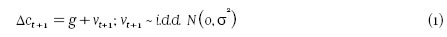We incorporate habit in consumption to capture intertemporal nonseparability. We assume that habit is external as in Campbell and Cochrane (1999). The habit process is conveniently expressed in terms of the consumption surplus ratio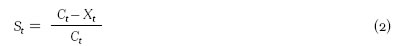The log consumption surplus ratio evolves as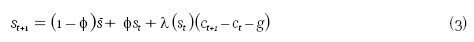where φ , g, and s¯ are parameters; and λ (st) is a sensitivity function given by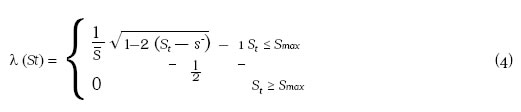which controls how S t + 1 and thus Xt+1 responds to contemporaneous consumption Ct +1.

In (4),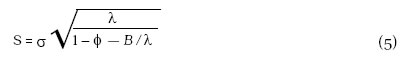and Smax is the value of St at which the square root in (5) runs into zero,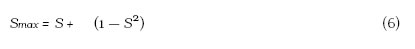S¯ and smax are the steady state and upper bound of the surplus consumption ratio, respectively. B = γ (1 – φ) – γ2 σ2 / S¯ 2 is the elasticity of the interest rate to deviations of the surplus consumption ratio from the steady state.

The specifications of the dynamic of consumption and the surplus consumption ratio imply that the habit process moves more slowly than consumption: consumption falls (increases) faster than the habit level, resulting in an instantaneous increase (decrease) in risk aversion. Therefore, risk aversion is countercyclical, which leads to countercyclical risk premia and expected returns. Countercyclical risk aversion means that risk aversion increases during recessions, when wealth is likely to be low.

3.2. The Household's Optimization Problem

The consumption and portfolio choice problem of a household is as follows. At the beginning of each period t , the household purchases Ct units of a consumption good at price Pt. There are J tradeable assets in the economy, indexed by j; and M monetary assets, indexed by m. In period t, the household invests Kjt units of wealth Wt in asset j and Kmt units in monetary asset m. The gross rates of return on asset j and monetary asset m in period t + 1 are R j,t +1 and Rm,t +1, respectively. The household's total saving in assets satisfies the intraperiod budget constraint: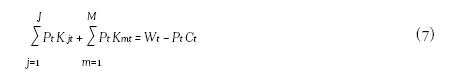The household's wealth in the following period satisfies the intertemporal budgetconstraint: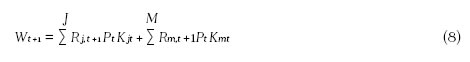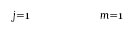From (7), (8) can be expressed as: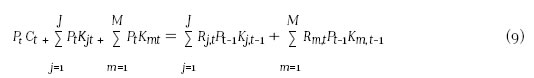To capture the demand for monetary services, we incorporate money in the house- hold's utility function. The household's intraperiod utility function is given by U (CtXt , Mt / Pt ); where Xt is the level of habit, Mt = M (mt) is the nominal amount of monetary holdings mt = [K1,t, K2,t , ..., KM,t ]0, and Pt is the general price level. Thus, household's utility is function of current private consumption, Ct, as well as current and past aggregate consumption, Xt. Since the habit is external, individual agents do not consider the effects of current consumption on future utility.

We further assume that the utility function is separable between consumption and real monetary holdings. Thus, the household intertemporal utility function is given by: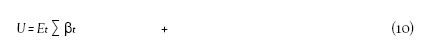Given the household's current level of wealth Wt , the household chooses consumption and saving {Ct, Mt , K1,t , K2,t , ..., KJ,t} to maximize its utility (10) subject to the constraint (9).

3.3. Marginal Utility and Asset Prices

Intratemporal marginal utility with respect to consumption Ct is given by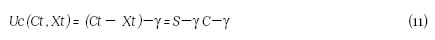Thus, the itertemporal marginal utility, or stochastic discount factor, is: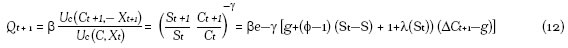Maximizing (10) subject to (9) yields: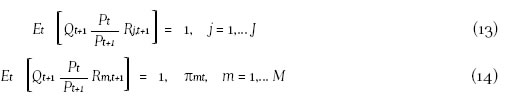where πmt = Uc (Ct, Xt)/Um (Km,t ) ≥ 0 is the intratemporal marginal rate of substitution between consumption and the monetary asset Kmt; and captures the user cost of money of the monetary asset Kmt, see Barnett and Wu (2005). Equations (13) and (14) are the pricing equations for tradable and monetary assets, respectively. Equation (13) is the fundamental equation of any dynamic asset pricing model. Equation (14) applies to any monetary asset providing liquidity services. Combining (13) and (14) we get: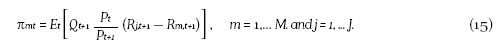Equation (15) shows that the user cost of any monetary asset m is given by the discounted difference between the return on a tradeable asset and the monetary asset m. The discount factor is stochastic and corresponds to the intertemporal marginal rate of substitution of consumption—which is the appropriate measure of risk for an individual who cares about consumption. During bad times, consumption is low and thus marginal utility is high. Assets that yield low returns in bad times are risky since they fail to deliver when investors need them the most. Thus, risky assets offer high expected returns to compensate investors for bearing risk. In contrast, assets that dowell in bad times hedge investors and thus offer low expected returns1.

If there exists a risk free asset providing monetary services, its risk free rate obeys: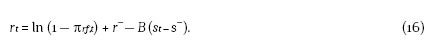where πr f, t ≥ 0 is the user cost of the risk free asset and r¯ = − lnβ + γ g – γ σ22 2S2 is the steady state (log)interest rate. If πr f, t = 0, the risk free asset provides no monetary services. The risk free interest rate is constant when B = 0 —the baseline case in Campbell and Cochrane (1999). If B 0, interest rates are low (high) in bad times and high (low) in good times (Verdelhan, 2010). It can be shown that B 0 implies a procyclical (countercyclical) real risk free interest rate and a downward (upward) sloping yield curve (Møller, 2009).

4. Estimation

Under the maintained assumption of separability between money and consumption, we can estimate the user cost of monetary assets in two steps. First, we estimate the model parameters using (13). Second, we compute the user cost of monetary assets, πmt , using (14) or (15). A one-step estimation is hampered by the unequal and sometimes shorter length of time series data on monetary assets compared with equity and interest rate data.

4.1. Model Parameters

We estimate the model parameters q= {λ, g, σ, S, β, φ} using the generalized method of moment (GMM) approach of Hansen and Singleton (1982). Unlike Campbell and Cochrane (1999), we allow for a time-varying risk free rate. Equations (1), (13), and (20) yield the following population moment conditions:

Moment conditions (17)-(20) lead to q orthogonality conditions of the form E [g (Xt, θ)|Zt] =0, where X represent data and Zt the conditioning information set at time t . Using a vector of instrumental variables Zt observed at t , these orthogonality conditions become E [g (Xt , θ)|Zt ] =0. GMM estimates the sample counterpart of these orthogonality conditions, gt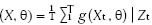by choosing q such that gT (θ) is a close as possible to zero. The GMM estimator is defined as follows: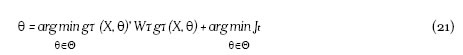where WT is a q x q positive semi-definite weighting matrix. The optimal choice of Wt is given by the inverse of the covariance matrix of the sample orthogonality conditions. The GMM estimator is consistent under relatively weak conditions—in large samples, the minimum of JT approximate the minimum of the population objective function.

We estimate θ using 2-step, k-step, and the continuously updating GMM estimators, Hansen, Heaton, and Yaron (1996). Compared to 2- and k-step optimal GMM estimators, the continuously updating estimator—when it converges— is more efficient, less biased, and has better small-sample properties, see Hansen et al. (1996), Donald and Newey (2000), and Antoine, Bonnal, and Renault (2007).

The estimation of the model parameters is numerically demanding since the surplus consumption ratio process in (3) is unobserved. To overcome this difficulty, we initialized the surplus consumption ratio at its steady state value, s¯, and estimate all the parameters jointly. For each iteration of the GMM estimation, the parameters affecting the surplus consumption ratio change and a new consumption ratio process is generated. The process continuous until (21) convergences. The estimation procedure needs to keep the parameters within their joint feasible space. Thus, we impose box and inequality constraints in our estimation —γ > 0, σ > 0, S¯ > 0, β > 0, φ > 0, and 1 – φ – B / – γ > 02.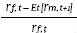To compute WT , we use Newey and West's (1987) heteroscedasticity and autocorrelation consistent (HAC) covariance matrix estimate with Andrews's (1991) automatic bandwidth selection using quadratic spectral and Tukey-Hanning kernels. We obtain an initial consistent estimator of WT in a one step GMM estimation using the identity matrix as the weighting matrix.

4.2. User Cost of Monetary Assets

After estimating the model parameters, we compute πmt using three alternative methods. First, using (13), Et [Qt +1] =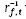, where r f, t is the real risk-free rate. Then, denoting real returns by rm and r j , (14) can be written as: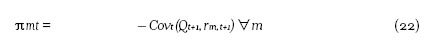Second, we can write (14) as: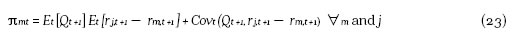The first method implies that the benchmark asset is the risk-free interest rate i the economy and the second implies that any risky asset can be used as the benchmark asset (Barnett and Wu, 2005). In theory, (22) and (23) should give identical results. Our empirical application shows that they differ but show a similar pattern over time.

In practice, (22) and (23) may yield πmt < 0. To avoid excessive negative user cost estimates, we consider a third method. We use the envelop approach of Barnett, Offenbacher and Spindt (1981) and Hancock (2005a,b) under which the benchmark rate equals the highest expected return among all monetary assets, rB,t = max {Et [r 1, t +1], ... Et [rM t +1]} for each t. Then, we compute πmt using rB,t instead of r f ,t in (22).

To operationalize (22) and (23), we need to estimate the expected values of Qt +1, r j,t +1, and rm,t +1 at time t. Following the literature, we use time series models to compute the one step-ahead forecast for these variables (see Elger and Binner, 2004). In addition, we use Engle (2002)'s dynamic conditional correlation model (DCC) to estimate the covariance terms in (22) and (23). The DCC model gives estimates of time-varying correlations, ρ tx, y, between any two variables x and y and estimates of their time-varying conditional variances σ2t,x and σ2t,y in a multi-GARCH (generalized autoregressive conditional heteroscedasticity) framework. Then, time-varying conditional covariances between any x and y can be computed as σtx,y = ptx,y σt, x σt, y.

5. Data

To estimate the model we need consumption, population, equity returns, and interest rate data. We use quarterly U.K. data for the period between 1965Q1 to 2011Q1. Thus, we have 185 observations for each time series. Consumption data comes from the Office for National Statistics (ONS). We measure consumption as seasonally adjusted households' expenditures on non-durables (UTIJ) and services (UTIN). We deflate consumption, interest rates, and asset returns data using the implicit price deflator for expenditures on non-durables and services. To compute per capita consumption, we use mid-1965 to mid-1974 U.K. resident population estimates from the CIA's World Fact Book and mid-1975 to mid-2010 from the ONS3.

We obtain equity returns from Datastream®. We use the total return index of the FTSE (All shares) and six economic sectors (industrial, oil and gas, materials, consumption goods, health care, and non-financial) to compute quarterly returns. As a proxy for the general level of interest rates, we use the interest rate on 3-months government bonds from the FRED® Economic Data published by the Federal Reserve Banks of St. Louis, U.S.A.

In addition, to test the robustness of our results, we compute the model parameters using value- weighted six Fama-French portfolios sorted by size and book-to-market value from 1980Q4 to 2010Q44. Since the Fama-French portfolio data are at a monthly frequency but consumption data are at a quarterly frequency, we aggregate monthly data to a quarterly frequency before estimation.

To compute the user cost of monetary assets, we need data on monetary aggregate components for the U.K. We consider the following components used in the computation of the Monetary Services Divisia Index: Interest-bearing banks sight deposits (1977Q1-2011Q1), interest-bearing bank time deposits (1977Q1-2011Q1), interest-bearing sight deposits at mutuals (1998Q4-2011Q1), interest-bearing sight deposits at mutuals (1998Q4-2011Q1), and cash individual saving accounts (ISA). (1999Q2-2011Q1). Definitions and data for each component are available at the Bank of England5.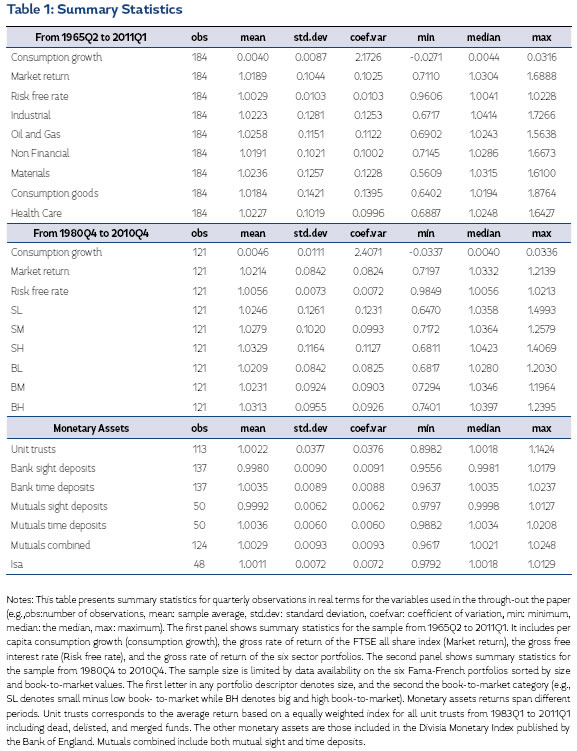We also consider monetary holdings at U.K. unit trusts. Unit trusts are openended investment funds offering some features akin to other monetary assets like bank deposits. For instance, unit trusts usually allow withdrawals within a period comparable to those of bank time deposits. Compared to bank deposits and most monetary assets, unit trusts carry higher risks. We use Datastream®to obtain datafor all U.K. unit trust funds from 1983Q1 to 2011Q1. We compute average returns including dead, delisted, and merged funds for all unit trust. Our dataset is limited since we cannot identify all funds by their objective strategy. Thus, we build a quarterly total return index giving equal weight to each fund.

Table 1 presents summary statistics for the variables we use in the estimation (e.g., obs:number of observations, mean: sample average, std.dev: standard deviation, coef.var: coefficient of variation, min: minimum, median: the median, max: maximum). All values are in real terms and are computed from quarterly observations. The first panel shows summary statistics for the sample from 1965Q2 to 2011Q1. In this sample, we include per capita consumption growth (consumption growth), the gross rate of return of the FTSE all share index (Market return), the gross free interest rate (Risk free rate), and the gross rate of return of the six sector portfolios. The second panel shows summary statistics for the sample from 1980Q4 to 2010Q4. The sample size is limited by data availability on the six Fama-French portfolios sorted by size and book-to-market values. The first letter in any portfolio descriptor denotes size, and the second the book-to-market category. For instance, SL denotes small minus low book-to-market while BH denotes big and high book-to-market.

6. Empirical Results

6.1. Model Parameter Estimates

In this subsection, we estimate the Campbell and Cochrane's model parameters θ = {γ, g, σ, S, β, φ} and the implied elasticity of the interest rate with respect to deviations from the steady state of the surplus consumption ratio, B. The estimates represent the set of parameters that minimize the pricing errors of the Euler equations (17)-(20).

We consider two sets of test assets. First, we use as test assets the FTSE all share returns, the risk free interest rate, and six sectors returns (industrial, oil and gas, materials, consumption goods, health care, and non-financial sectors). Second, we use the six Fama-French portfolios sorted by size and book-to-market value, the risk free interest rate, and a weighted-value market return as described in Gregory et al. (2009). We include in the conditional information set the lag of the risk free interest rate, the market return, and consumption growth for a total of forty-moment conditions. The sample period for the six sector portfolios extends from March 1965 to March 2011 and for the six Fama-Frech portfolios from December 1980 to December 2010.

Table 2 reports the estimated values for the structural parameters and the implied B parameter. We present results using three alternative methods: two-steps GMM (2-steps), continuously updating estimator GMM (CUE), and iterative GMM (ITER). In general, the results across the three methods are comparable. We emphasize the results from the continuously updating GMM method since it has better small sample statistical properties. Following Campbell and Cochrane's, we use γ = φ = 0.965, b = 0.971 and S¯ = 0.057 as starting values. For g¯ and s, we use their sample estimates presented in Table 1.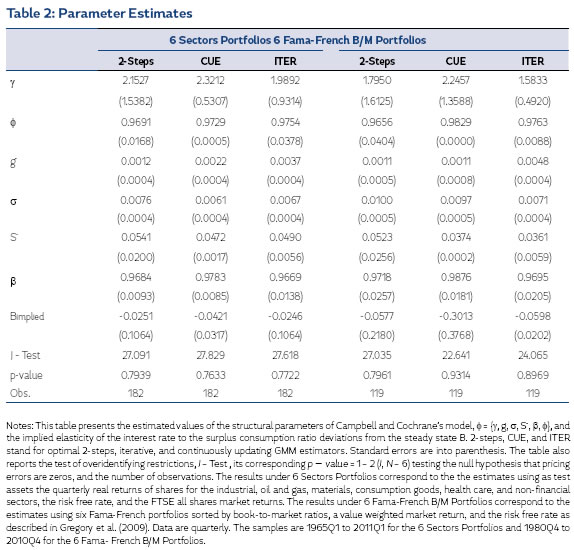To compute the optimal weighting matrix, we estimate the HAC covariance matrix using the quadratic spectral kernel for the 2-steps-GMM and ITER-GMM methods since it has better statistical properties, Andrews (1991). We use the Tukey-Hanning kernel for the CU-GMM method since it is numerically more stable and has statistical properties comparable to those of the quadratic spectral kernel. We compute standard errors using the estimated HAC covariance matrix. For the implied parameter B, we use the delta method to compute its standard errors.

The estimated structural parameters seem reasonable and in line with the literature. The parameter estimates for g vary from 1.58 to 2.32 with relatively high standard errors. Campbell and Cochrane use g = 2 for their post-war period U.S. application.

The persistence parameter estimates, Φ, vary from 0.966 to 0.986. For the six sector portfolios case, the estimates of the mean consumption growth, g¯ γ, range from 0.22% to 0.37%. In the data, g¯ = 0.39%. This indicates a highly persistent surplus consumption process in line with the findings in Tallarini and Zhang (2005) and Verdelhan (2010). The estimates of the volatility of consumption growth, s, range from 0.61% to 0.67%. In the data, σ = 0.86%. Their standard errors are very small. For the six Fama-French portfolio case, mean consumption growth estimates are below their sample estimate of 0.48% while its volatility estimates are close to its sample estimate of 1.05%.

Average surplus consumption ratio estimates, S¯, are between 3.71% and 5.23%, which indicates that the habit level of consumption is between 94.77% and 96.29%. The estimations for B, the elasticity of interest rates with respect to deviations from the steady state consumption ratio, are negative but their standard errors indicate that they are statistically insignificant, which suggest a constant interest rate. This result is consistent with Campbell and Cochrane who show that adding interest rate variation through B has very little effect in their results. The estimates of the subjective discount factor are around 0.97 which implies a subjective discount rate of around 3% per quarter.

The J-Test values and their associated p-values in Table 2 indicate that the CC model is not rejected in our two samples. The differing results between the 2-steps and iterative GMM estimators suggest that weak identification of the model parameters maybe a concern (Stock, Wright and Yogo, 2002). Stock and Wright (2000) show that the continuously updating GMM estimator seems to be partially robust to weak identification. They propose to use a robust overidentiying test in the presence of weak identification, the S-Test. In our case the J-Test presented in Table 2 for the CUE estimator coincides with the S-Test. Thus, even though we cannot rule out weak identification of the model parameters, the CUE GMM results seems to be robust to such a problem. In the following subsection, we use the CUE GMM results to estimate the user cost of monetary assets.

6.2. Risk-adjusted User Cost of Monetary Assets

After estimating the structural parameters of the CC model, we are in a position to estimate the the risk adjustment and the user cost of monetary assets. Equation (22) is a risk-adjusted user cost formula derived under the assumption that there exists a risk free benchmark rate, in this case the risk-free interest rate. This formula depends on the expected return of each monetary asset as well as on an estimate of the covariance between each monetary asset return and the stochastic discount factor (SDF).

The first term in (22), (r f ,tEt [rm,t +1])/r f ,t , corresponds to the certainty-equivalent user cost of each monetary asset m. The second term, – Covt (Qt +1, rm,t +1), is the risk- adjustment to the certainty- equivalent user cost. When the risk-adjustment is large, Divisia Monetary Indexes using the certainty-equivalent user cost will be biased. Table 3 presents summary statistics of the risk-adjustment estimates for each monetary asset over their respective sample period and Figure 1 depicts their behavior over time.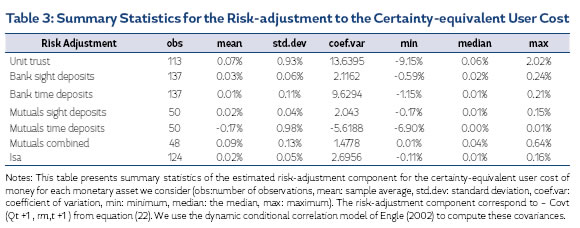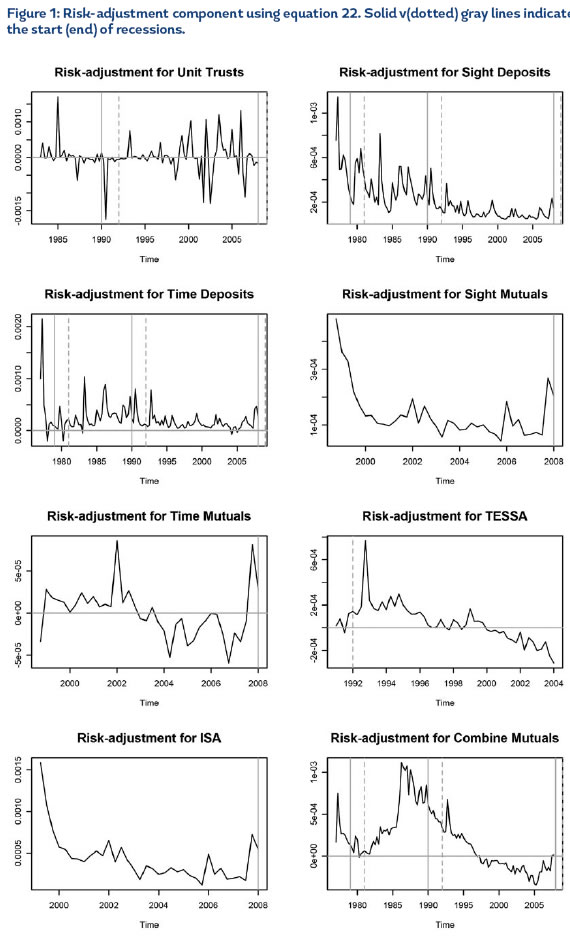The mean and the median of the risk-adjustment estimates for each monetary assets are positive but close to zero, indicating that the risk-adjustment can be neglected in the construction of Divisia monetary aggregates. Even for the riskiest monetary asset, unit trust funds, the risk adjustment is negligible. Our results are consistent with Barnett et al. (1997) who consider time separable preferences.

The mean and the median of the risk-adjustment estimates for each monetary assets are positive but close to zero, indicating that the risk-adjustment can be neglected in the construction of Divisia monetary aggregates. Even for the riskiest monetary asset, unit trust funds, the risk adjustment is negligible. Our results are consistent with Barnett et al. (1997) who consider time separable preferences.

Barnett and Wu (2005) show that under uncertainty, (14) implies that any non monetary asset could be used as the benchmark asset in the estimation the user cost of monetary assets. In Table 4, we report the user cost estimates taking the risk-free rate (first panel) and the market return (second panel) as the benchmark rates. Figures 2 and 3 depict their behavior over time. The results indicate that for all monetary asset there are negative user costs. This is not a theoretical problem of the CC model, but a feature of the data at hand. For instance, if the yield curve is inverted such that the return on short-term monetary assets is greater than the return on a long-term non-monetary asset, the user cost may be negative. This is a violation of the assumption that monetary assets offering higher levels of monetary services should yield lower return rates.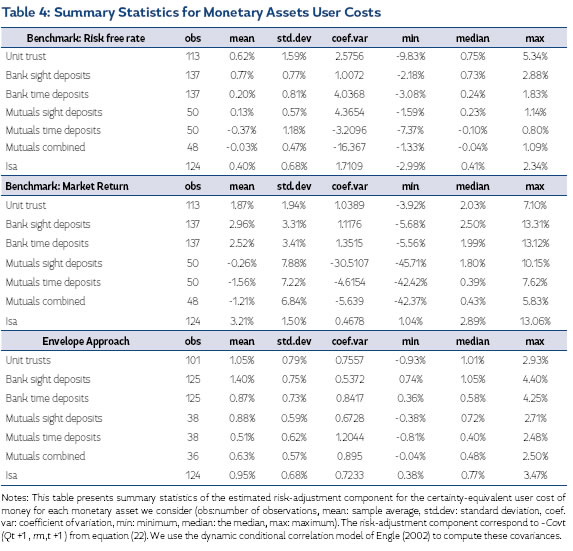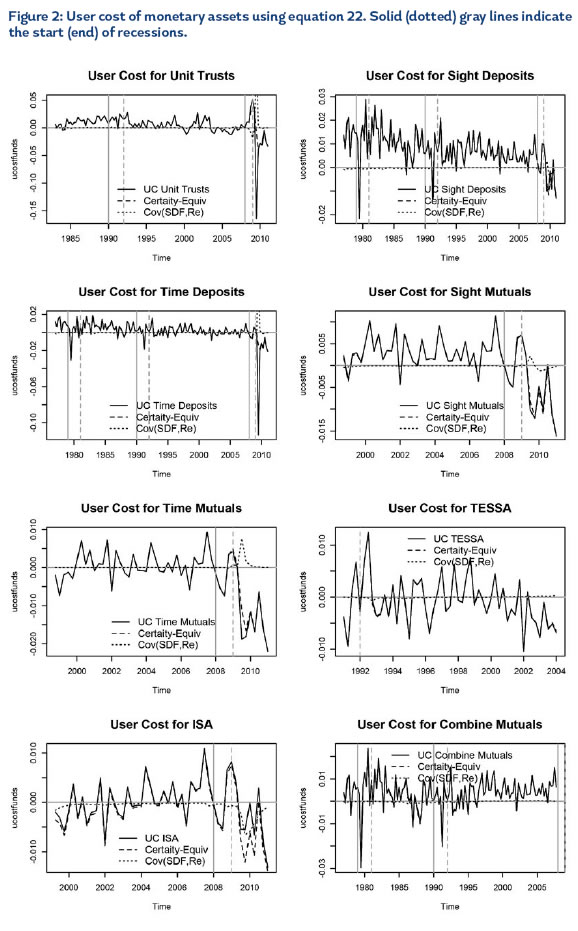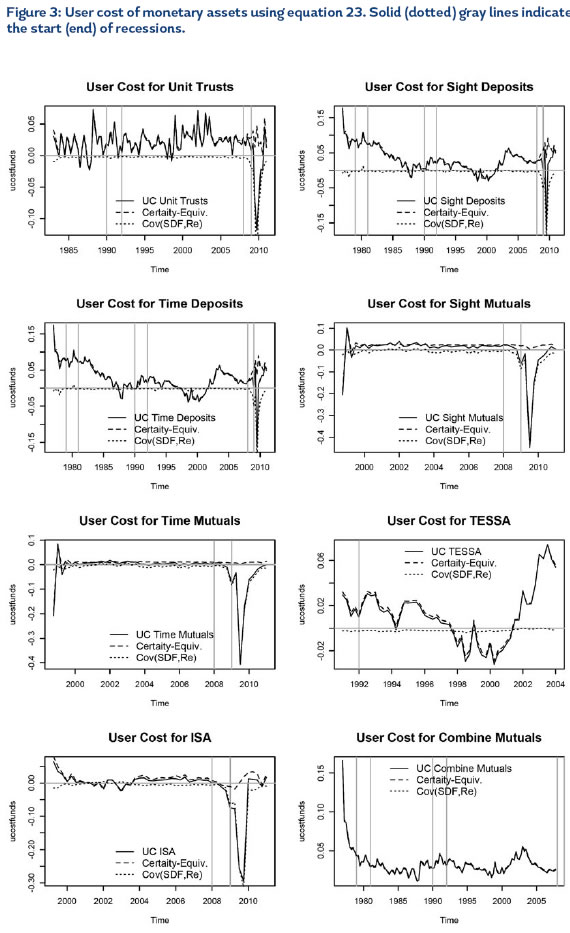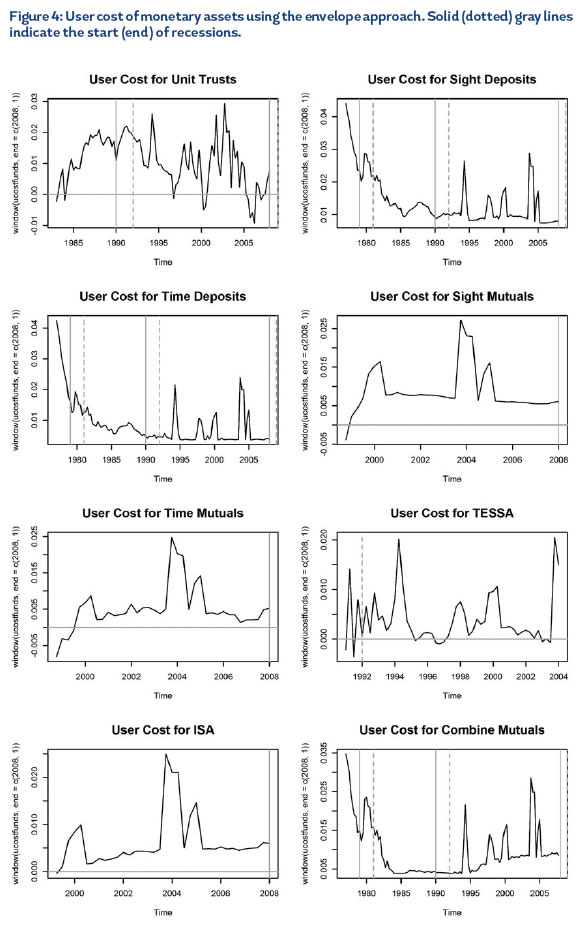Negative user costs are a common problem in the construction of Divisia aggregates. To solve the problem, researchers have to make further assumptions or arbitrary adjustments to make them conform with theory. For instance, researchers at the Bank of England and the U.S. Federal Reserve resolve this problem by defining the benchmark asset to be the asset with the highest return, regardless of market liquidity or time to maturity, see Hancock (2005a,b) , Anderson and Buol (2005), Anderson and Jones (2011). This is known as the envelope approach. According with this method the benchmark rate corresponds to the highest expected return among all monetary assets, rB, t = max {Et [r1,t +1], ... Et [rM,t +1]} for each t.

We implement the envelope approach by computing πmt using rB,t instead of r f ,t in (22). Following the literature, we add a liquidity premium of 144 basis points to the benchmark rate to avoid negative user costs, see Anderson and Jones (2011). The third panel of Table 4 present the results and Figure 4 depict them over time. We exclude data from 2008 onward, since due to the collapse of interest rates after the recent U.S. financial crisis, most user cost become negative. As expected, the mean user cost estimates for each monetary assets are positive. However, note that for unit trusts, there are some negative values, reflecting the very negative results for this funds during those periods.

7. Conclusions

Barnett et al. (1997) show that the risk-adjustment to the certainty-equivalent user cost of money is negligible. Barnett and Wu (2005) hypothesize, however, that Barnett et al. (1997) approach may underestimate the magnitude of the risk-adjustment since they do not consider time non-separable preferences. Using the habit-based asset pricing model of Campbell and Cochrane (1999) (CC model), we find that under time non-separable preferences the risk adjustment user cost is also negligible. Therefore, the construction of Divisia monetary aggregates without accounting for risk are unlikely to be affected.

We use U.K. data from 1965Q1 to 2011Q1 to estimate the CC model using to sets of test assets. First, we use a market portfolio and six industrial sectors portfolios. Second, we test the model using six Fama-French portfolios sorted by size and book- to-market values from 1980Q4 to 2010Q4. Our results indicate that the CC model is not rejected by the data in the sense that pricing errors are small. The estimated parameters are reasonable and in line with the literature.

Using the parameter estimates from the CC model, we estimate estimate time and asset specific risk-adjustment to the certainty equivalent user cost of monetary assets included in the U.K. Divisia index. We also include unit trusts as a potential risky monetary asset. Using the dynamic conditional correlation (DCC) model of Engle (2002), we find that the average risk-adjustments for most monetary assets is positive but their magnitudes are too low to affect the construction of Divisia monetary aggregates.

Our results have two important implications for the construction of monetary indexes. First, researchers may dispense from risk-adjusting the user cost of money to construct such indexes. Despite their theoretical appeal, our empirical results show that the risk adjustment for risky monetary assets is negligible. Second, choosing a benchmark risky asset does matter for adjusting the user cost in empirical applications. Thus, the theoretical appeal of risk adjusting the user cost of risky monetary assets is lost since there are numerous benchmark assets to choose from. Any choice would be arbitrary and will likely lead to different results.

* Acknowledge financial support from the Colombian Fulbright Commission, the Colombian Administrative Department of Science, Technology and Innovation (Colciencias), and EAFIT University.

1 Our modeling strategy takes as given that monetary assets offer monetary services—households value monetary assets for their liquidity and for been claims to streams of consumption goods. However, equation (15) can also be derived from micro-founded models in which monetary assets are useful in facilitating exchange as in Lagos (2010) and Lagos (2011).

2 We use the constraint nonlinear optimization algorithm of Powell (1994) and available from the NLopt free-open-source library developed by Steven G. Johnson (http://ab-initio.mit.edu/ nlopt).

3 We interpolate annual population data to a quarterly frequency using a seasonal Kalman filter.

4 Available at http://xfi.exeter.ac.uk/researchandpublications/portfoliosandfactors/index.php. See Gregory, Tharyan and Huang (2009) for details.

References

Abel, A. B., May 1990. Asset Pricing under Habit Formation and Catching Up with the Joneses. American Economic Review (Papers and Proceedings) 80 (2), 38–42.

Anderson, R., Buol, J., 2005. Revisions to user costs for the Federal Reserve Bank of St. Louis monetary services indices. Review Federal Reserve Bank of Saint Louis 87 (6), 735.

Anderson, R., Jones, B., 2011. A comprehensive revision of the US monetary services (Divisia) indexes. Federal Reserve Bank of St. Louis Review 93 (5), 235–59.

Andrews, D., 1991. Heteroskedasticity and autocorrelation consistent covariance matrix estimation. Econometrica: Journal of the Econometric Society, 817–858.

Antoine, B., Bonnal, H., Renault, E., 2007. On the efficient use of the informational content of estimating equations: Implied probabilities and Euclidean empirical likelihood. Journal of Econometrics 138 (2), 461 – 487.

Barnett, W., 1978. The user cost of money. Economics Letters 1 (2), 145–149.

Barnett, W., Offenbacher, E., Spindt, P., 1981. New Concepts of Aggregated Money. The Journal of Finance 36 (2), 497–505.

Barnett, W. A., Liu, Y., Jensen, M., June 1997. Capm Risk Adjustment For Exact Aggregation Over Financial Assets. Macroeconomic Dynamics 1 (02), 485–512.

Barnett, W. A., Wu, S., 2005. On user costs of risky monetary assets. Annals of Finance 1 (1), 35–50. Boldrin, M., Christiano, L. J., Fisher, J. D. M., 2001. Habit Persistence, Asset Returns, and the Business Cycle. The American Economic Review 91 (1), 149–166.

Buraschi, A., Jiltsov, A., 2007. Habit Formation and Macroeconomic Models of the Term Structure of Interest Rates. Journal of Finance 62 (6), 3009 – 3063.

Campbell, J. Y., Cochrane, J. H., Apr. 1999. By Force of Habit: A Consumption-Based Explanation of Aggregate Stock Market Behavior. Journal of Political Economy 107 (2), 205–251.

Chen, X., Ludvigson, S. C., 2009. Land of addicts? an empirical investigation of habit-based asset pricing models. Journal of Applied Econometrics 24 (7), 1057–1093.

Constantinides, G. M., Jun. 1990. Habit Formation: A Resolution of the Equity Premium Puzzle. Journal of Political Economy 98 (3), 519–543.

Donald, S. G., Newey, W. K., 2000. A jackknife interpretation of the continuous updating estimator. Economics Letters 67 (3), 239–243.

Elger, T., Binner, J., 2004. The UK Household Sector Demand for Risky Money. The B.E. Journal of Macroeconomics 4 (1), 1–22.

Engle, R., 2002. Dynamic conditional correlation. Journal of Business & Economic Statistics 20 (3), 339–350.

Epstein, L. G., Zin, S. E., Apr. 1991. Substitution, Risk Aversion, and the Temporal Behavior of Consumption Growth and Asset Returns II: An Empirical Analysis. Journal of Political Economy 9 (2), 263–286.

Fuhrer, J., 2000. Habit formation in consumption and its implications for monetary-policy models. American Economic Review, 367–390.

Gregory, A., Tharyan, R., Huang, A., 2009. The Fama-French and momentum portfolios and factors in the UK. University of Exeter Business School, Xfi Centre for Finance and Investment Paper (09/05). Hancock, M., 2005a. A new measure of Divisia money. Bank of England Monetary & Financial Statistics, 13–14.

Hancock, M., 2005b. Divisia money. Bank of England Quarterly Bulletin, Spring.

Hansen, L. P., Heaton, J., Yaron, A., 1996. Finite-Sample Properties of Some Alternative GMM Estimators. Journal of Business & Economic Statistics 14 (3), 262–280.

Hansen, L. P., Singleton, K. J., September 1982. Generalized Instrumental Variables Estimation of Nonlinear Rational Expectations Models. Econometrica 50 (5), 1269–86.

Hyde, S., Sherif, M., 2005a. Consumption Asset Pricing Models: Evidence from the UK. The Manchester School 73 (3), 343–363.

Hyde, S., Sherif, M., 2005b. DonSt break the habit: structural stability tests of consumption asset pricing models in the UK. Applied Economics Letters 12 (5), 289–296.

Jones, B. E., Stracca, L., 2008. Does money matter in the IS curve? The case of the U.K. The Manchester School 76, 58–84.

Krishnamurthy, A., Vissing-Jorgensen, A., 2012. The aggregate demand for treasury debt. Journal of Political Economy 120 (2), 233–267.

Lagos, R., 2010. Asset prices and liquidity in an exchange economy. Journal of Monetary Economics 57 (8), 913–930.

Lagos, R., 2011. Asset prices, liquidity, and monetary policy in an exchange economy. Journal of Money, Credit and Banking 43 (s2), 521–552.

Møller, S., 2009. Habit persistence: explaining cross-sectional variation in returns and time-varying expected returns. Journal of Empirical Finance 16 (4), 525–536.

Newey, W., West, K., 1987. A simple, positive semi-definite, heteroskedasticity and autocorrelation consistent covariance matrix. Econometrica: Journal of the Econometric Society, 703–708.

Powell, M., 1994. A direct search optimization method that models the objective and constraint functions by linear interpolation. Advances in optimization and numerical analysis 7, 51–67.

Stock, J., Wright, J., Yogo, M., 2002. A survey of weak instruments and weak identification in generalized method of moments. Journal of Business & Economic Statistics 20 (4), 518–529.

Stock, J. H., Wright, J. H., 2000. GMM with Weak Identification. Econometrica 68 (5), 1055–1096.

Tallarini, T. D., Zhang, H. H., 2005. External Habit and the Cyclicality of Expected Stock Returns. The Journal of Business 78 (3), 1023–1048.

Verdelhan, A., 2010. A Habit-Based Explanation of the Exchange Rate Risk Premium. The Journal of Finance 65 (1), 123–146.

Wachter, J. A., 2006. A consumption-based model of the term structure of interest rates. Journal of Financial Economics 79 (2), 365–399.

Yogo, M., 2006. A Consumption-Based Explanation of Expected St0ock Returns. The Journal of Finance 61 (2), 539–580.